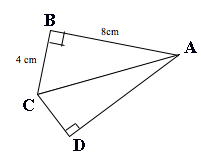SEARCH HOMEMath Central Quandaries & QueriesHello, I'm Syed from Bangladesh. Please help me out! It is killing me. The question is, find the perimeter of the figure. I can't find the perimeter. I am enclosing the figure as an attachment. Please, HELP ASAP!Hi Syed,

I reproduced the diagram you sent and added the letters $A, B, C, \mbox{ and }D.$Is this all you know? The perimeter is $8 + 4 + |CD| + |DA|$ and since triangle $ABC$ is a right triangle you can use Pythagoras theorem to show that $|CA| = \sqrt{8^2 + 4^2} = \sqrt{80}.$ From this information you can't determine $|CD|$ or $|DA|,$ all that you can say is that $|CD|^2 + |DA|^2 = 80^2.$

PennyMath Central is supported by the University of Regina and The Pacific Institute for the Mathematical Sciences.